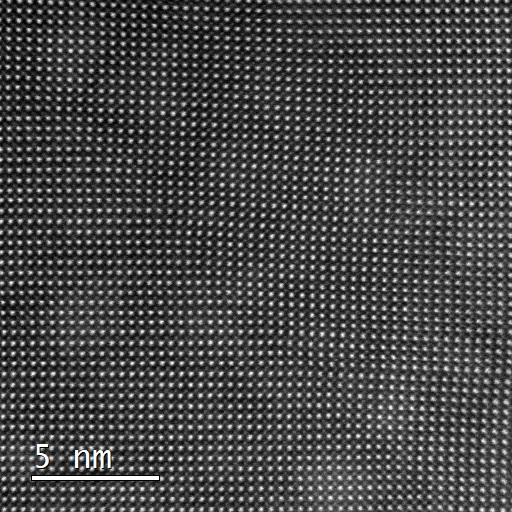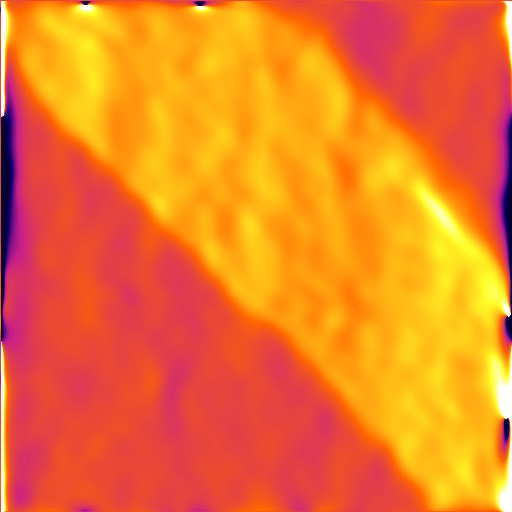# Home

## AboutFigure Example ADF image of PbZr0.2Ti0.8O3 and a corresponding $$e_{xx}$$ distortion map.

Strain++ is an open source program used to measure strain from high resolution transmission $test$ electron microscope (TEM) images. Strain is measured using the geometric phase analysis (GPA) algorithm as detailed in Martin Hÿtch’s paper:

Hÿtch, M. J., Snoeck, E. & Kilaas, R. Quantitative measurement of displacement and strain fields from HREM micrographs. Ultramicroscopy 74, 131–146 (1998)

GPA is a Fourier space technique and is therefore more robust to noise than real space techniques, making it a great tool for quick and easy strain analysis.

## Libraries

The external libraries used are listed here with their uses and licenses

• Eigen - matrices and linear regression (MPL2)
• QCustomPlot - plotting (GPLv3)
• Qt - GUI (GPLv3)
• FFTW - Fourier transforms (GPLv2)
• LibTIFF - TIFF input/output (BSD-like)